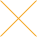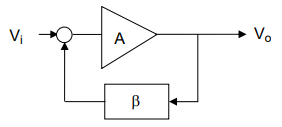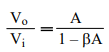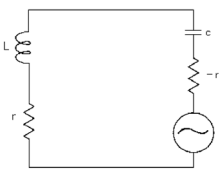Our website uses cookies. By continuing to use this website, you consent to cookies being used.
English
English

# Understanding VCO Concepts

## OSCILLATOR FUNDAMENTALS

An oscillator circuit can be modeled as shown in Figure 1 as the combination of an amplifier with gain A (jω) and a feedback network (jω), having frequency-dependent open-loop gain H (jω) = ßA.Figure 1: An oscillator may be modeled as the combination of an amplifier and a feedback loop

The general expression for closed-loop gainis which states that the system will oscillate, provided ßA = 1. At the frequency of oscillation, the total phase shift around the loop must be 360 degrees, and the magnitude of the closed loop gain must be unity. A common emitter circuit provides 180 degree phase shift. If the circuit is used with feedback from collector to base, the feedback circuit must provide additional 180 degree phase shift. If a common base circuit is used, there is no phase shift between the emitter and collector signals, and the feedback circuit must provide either 0 degree or full 360 degree phase shift.

Although the model shown in Figure 1 can be used to analyze and determine the necessary and sufficient conditions for oscillation, it is easier to use the model shown in Figure 2 where the analysis is performed in terms of a negative resistance concept. This is based on the principle that a resonator such as an inductor-capacitor tuned circuit, once excited, will oscillate continuously if no resistive element is present to dissipate the energy. It is the function of the amplifier to generate the negative resistance and maintain oscillation by supplying an amount of energy equal to that dissipated. The selection of the circuit topology is dictated by several factors:

1. Frequency of oscillation
2. Tuning range
3. Choice of transistor, and
4. Type of resonatorFigure 2: An oscillator may be modeled using a negative resistance concept

## EXAMPLES OF OSCILLATOR DESIGN

A bipolar transistor with capacitances between the base and emitter, and the emitter and ground, can be used to generate a negative resistance. Examples of these circuits are shown in Figure 3.

An inductive resonator may be used in any parallel resonant oscillator circuit. The signal is generated at the frequency where the inductor resonates with the load capacitance. The circuits of Figure 3 are preferred for high stability oscillator circuits because of the ease with which the resonator may be isolated from the load, which is in the collector circuit. In a VCO the variable capacitor is implemented using a varactor diode. The Clapp circuit has the advantage of better constancy of feedback because the ratio of C1 to C2 does not vary over the tuning range.

Consider the more detailed schematic diagram in Figure 4a, and the equivalent circuit of its resonator in Figure 4b that is used to derive the design equations below.

The input impedance looking into the part of the circuit to the right of the dashed line in Figure 4b is given by:

The quantity -(gm2 C1C2) is negative indicating a negative resistive component. Therefore, in order to maintain oscillation we must have

where r is the series resistance of the resonator. The frequency of oscillation is given by

The ratio C1/C2 is selected to be greater than 1 so that the circuit has sufficient loop gain for start-up condition at the lowest operating temperature. A VCO is implemented by replacing a fixed capacitor such as C3 with a varactor.

In another circuit that can generate a negative resistance, Figure 5 shows a bipolar transistor with an inductive reactance between the base and the ground. This common-base topology is preferred at higher frequencies. Adding an inductive resonator and a variable capacitor to form a tank circuit as shown in Figure 6 completes the oscillator.

## TUNING SENSITIVITY CHARACTERISTICS

There are two basic types of varactors: abrupt and hyperabrupt. The abrupt tuning diodes have very high Q and will operate over a very wide tuning voltage range (0V to 60V). The abrupt tuning diode provides the best phase noise performance because of its high quality factor.

Hyperabrupt tuning diodes, having capacitance inversely proportional to the square of the voltage, will provide a much more linear tuning characteristic than the abrupt diodes. These are the best choice for wide band tuning VCOs. An octave frequency range can be covered with less than 20V tuning voltage range. Their disadvantage is that they have a much lower Q and therefore provide a phase noise characteristic higher than that provided by the abrupt diodes.

For a varactor diode, the capacitance is related to the bias voltage by the following equation:

where A is a constant, VR is the applied reverse bias voltage, and Ф is the built-in potential which is 0.7V for silicon diodes and 1.2V for gallium arsenide diodes. For the analysis that follows, we may write

where A is the capacitance of the diode when V is one volt and n is a number between 0.3 and 0.6, but can be as high as 2 for a hyperabrupt junction. The tank circuit of a typical VCO has a parallel tuned circuit consisting of an inductance L, a fixed capacitance Cf, and a varactor diode C. The frequency of oscillation can be written as

Let ωo be the angular frequency of the unmodulated carrier, and let Vo and Co be the corresponding values of Vo and Co. Then from Equation (3) we have

If Vo is modulated by a small voltage ΔV, the carrier will be deviated by a small frequency difference Δω .